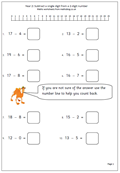# Calculating in Year 2In Year 2 children will be calculating using the addition, subtraction, multiplication and division symbols. Their understanding of addition will develop as they begin to add a 1-digit number to a 2-digit number as well as beginning to answer written addition questions. (This is not the same as using written methods.) Developing efficient mental methods of addition and subtraction are still the most important aspects here, as written methods, apart from jottings, should not yet be used.

Subtraction is much easier once it is realised that it is the inverse (or opposite) of addition. Many subtraction questions can be answered by adding on, but children are expected to know subtraction facts to at least 10 (eg know that 9 – 4 = 5). Knowing these facts is just as important as knowing ‘tables’. They will also be working with 2-digit numbers, especially multiples of 10.

There is a good selection of addition and subtraction worksheets on the site in our Year 2 calculating section as well as a growing number of multiplication and division pages. Multiplication is seen as repeated addition and the 2x, 5x and 10x tables will be learned during the year.

As well as in the Calculating section further worksheets can be found in Knowing Number Facts and our Four Rules section.

Go to Year 2 calculating worksheets